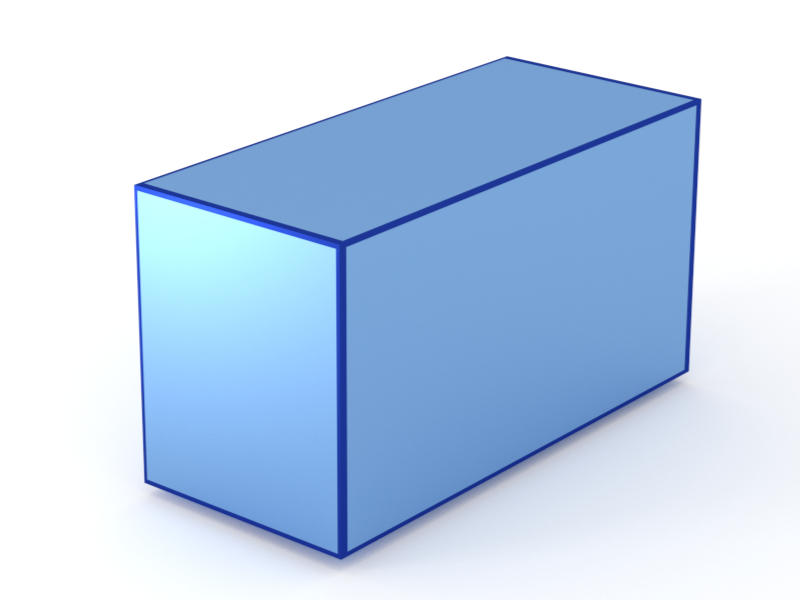### Box

A rectangular cuboid.#### Constructor

Box(L, W, H)


Parameters:

• L, length of the base
• W, width of the base
• H, height

#### Usage

As for any other Form factor.

#### Implementation

Class Box inherits from the interface class IFormFactor .

Form factor is computed as $$F(\mathbf{q})=LWH \space \exp\Big(iq_{z}\dfrac{H}{2}\Big) \space \text{sinc}\Big(q_{x}\dfrac{L}{2}\Big) \space \text{sinc}\Big(q_{y}\dfrac{W}{2}\Big) \space \text{sinc}\Big(q_{z}\dfrac{H}{2}\Big).$$

Volume has been validated against $$V=LWH.$$

More general:

More special:

• Bar, if elongated in L or W direction.

Modified cubes:

#### Example

Scattering by uncorrelated, oriented boxes for horizontal incidence. Rotation around $z$ axis:Generated by Examples/ff/Box.py .

#### History

Agrees with “Box” form factor of IsGISAXS [manual, Eq. 2.37; Renaud 2009, Eq. 214], except for factors $1/2$ in the definition of parameters $L,\space W,\space H$.